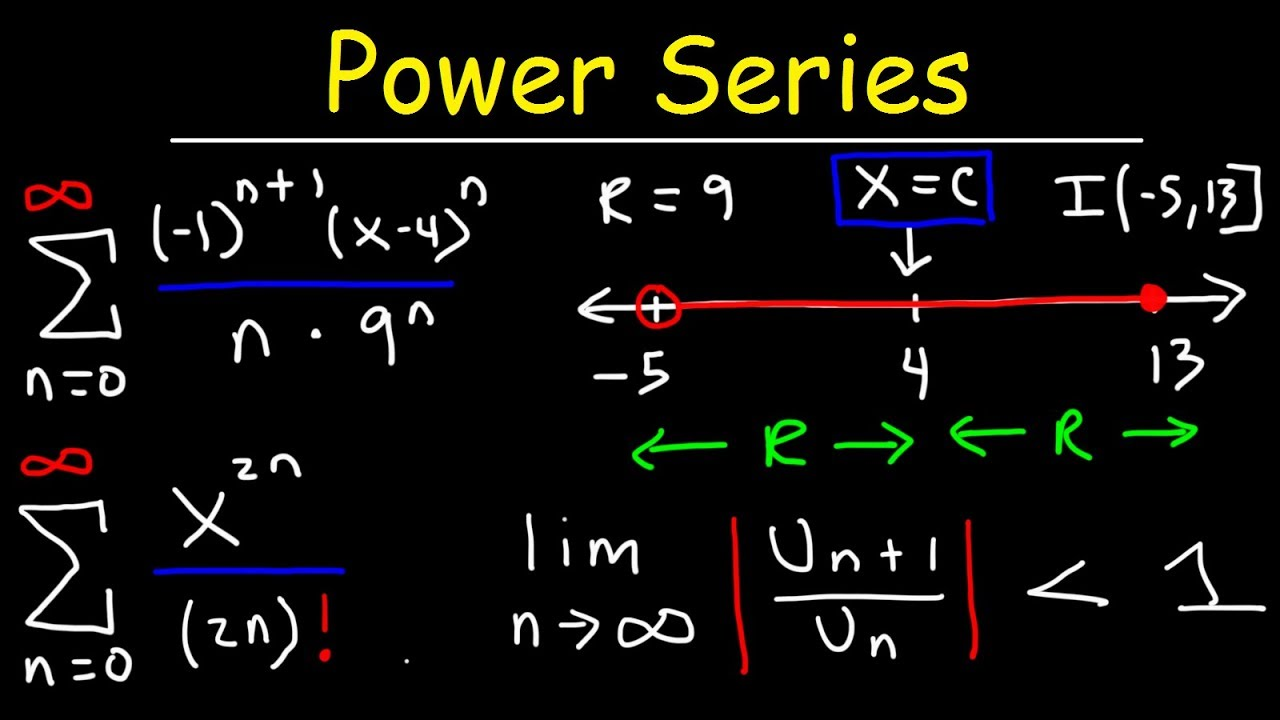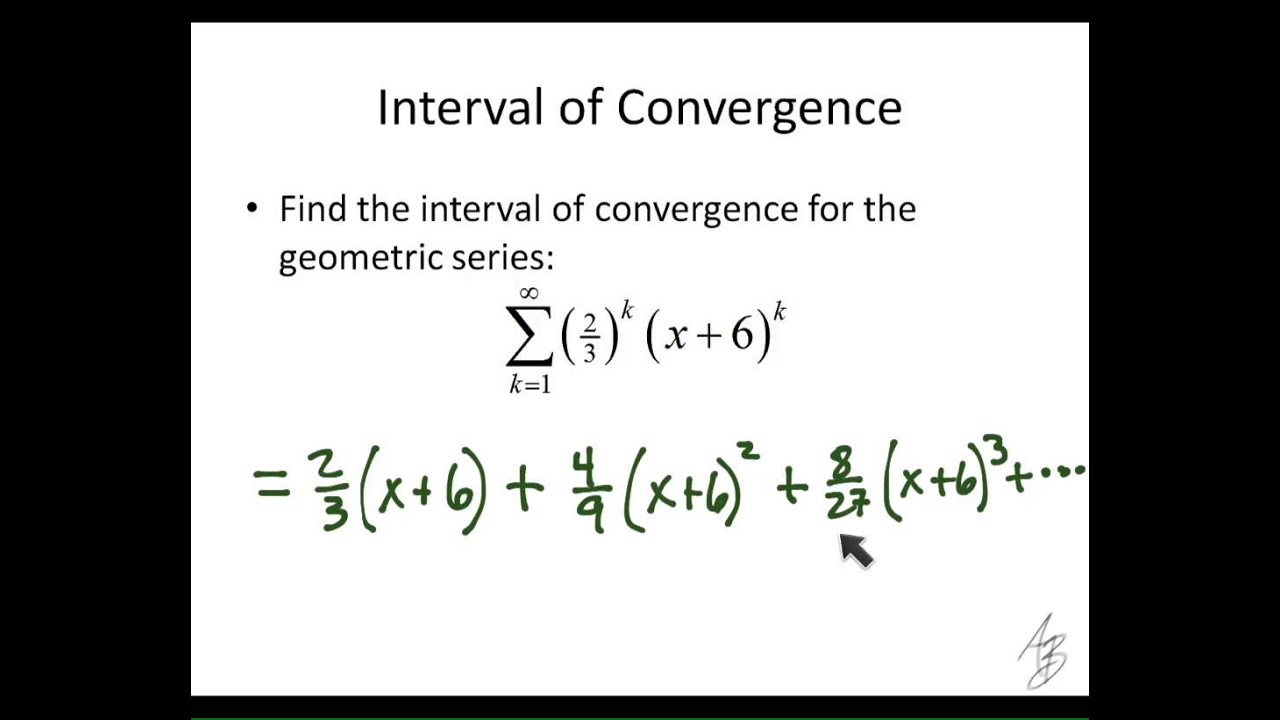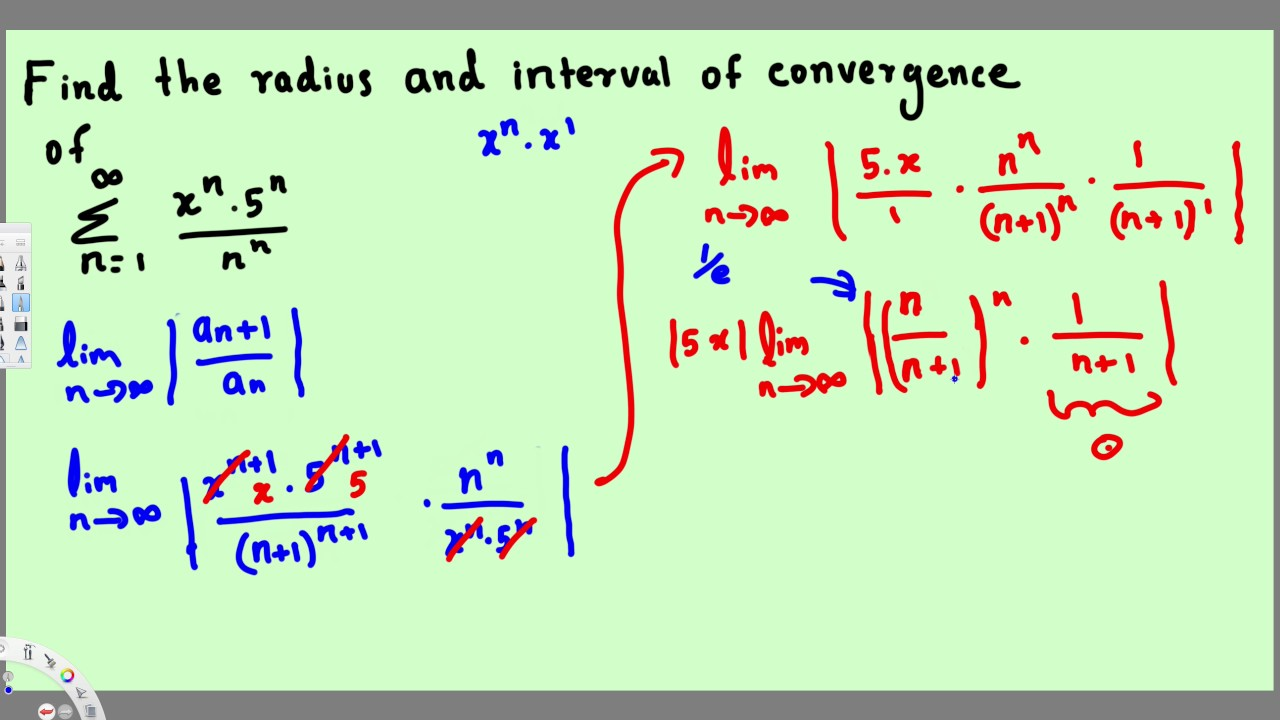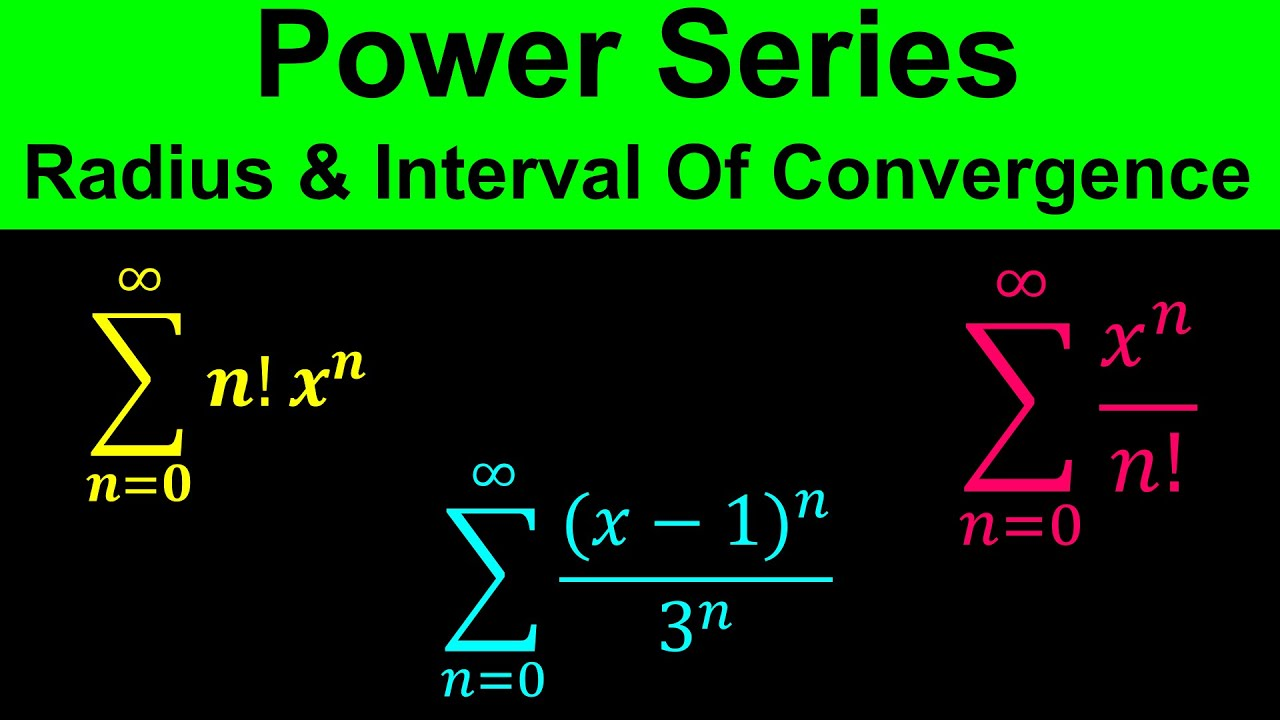# Interval of convergence calculator- meaning, use, series

In today’s world, we can’t imagine a day without calculations. From maintaining accounts to space travels, everything requires calculations. But as science is developing, the need for new and advanced calculators is exponentially increasing as well. In this article, we will learn everything about the interval of convergence calculator, and how to calculate it.

## What is an Interval of Convergence calculatorThe first thing that should come to your mind is exactly is an interval of convergence is? The interval of convergence of a series represents the set of values for which the series converges. Moreover, an interval associates with a given power series in such a way that the series diverges for all values of the values outside the interval and converges for all values inside it.

### Series Interval of convergence calculatorThe interval of convergence can be represented as the interval in which a particular series shows absolute convergence for a power series. It is expressed in interval notation. For example, suppose there is a series that shows the convergence between 2 which is inclusive, and 8 (exclusive). As a result, we can write it as (2, 8) or as 2 smaller than x and x smaller than 8.

In other words, power series shows an infinite series of the form:

∑n=0∞cn(x–a)n

Where cn represents a coefficient that varies with n. In addition, the series works as a function of x where the terms vary with the nth term of the series.

Furthermore, a power series can add an infinite number of successive terms. The sum of the terms can either be infinite or finite.

The series is converging if the sum of terms is a finite number. The series is diverging if the sum of the terms is infinite. By solving for the interval of convergence, we find the range of values for x in |x – a| less than R such that the series converges.

### Interval of Convergence calculator useNeedless to say, computers have an edge over humans at certain types of calculations but face difficulties performing complex  calculations. Therefore, we can successfully program an otherwise incapable computer to indirectly find solutions via the use of a power series if we can learn how to find the interval of convergence.

In order to evaluate ex with a large exponent, a calculator’s computer has to multiply large, messy numbers by large, messy numbers again and again. The computers generally store floating-point numbers and create round-off errors. As a result, this process can a considerable amount of time and even after that provide you with an inaccurate answer..

Luckily, the power series f(x) = xn⁄n! represents the expression ex when carried out to many terms. We find that it is ∞ less than x less than ∞  when we check the interval of convergence for the power series. In simple terms, it means the power series will converge everywhere and can be used for ex with all possible input values of x. If we are able to programme this routine into a computer, we will be able to enable it to accurately solve for the value of ex with any value of x. This is just one small example of how the use for the interval of convergence can affect our technology.

### Interval of Convergence calculator Power SeriesWhen we try to solve for convergence of a power series, we get multiple test options to choose from. These are of two types: the very common ratio test and root test.

In the ratio test, we use a ratio of the power series and a modified (n + 1) version of itself to solve x. We need to make sure that it follows the convergence criteria. The formula for the ratio test is:

Convergence when L is less than 1,

L=limn→∞|an+1an|

Where “an” represents the power series and “an + 1” represents the power series with all terms n replaced with n + 1.

The first step of ratio test is to put the original and modified versions of the power series into their respective places in the formula. Then, notice the limit as n tends to infinity. By plugging infinity in for n, the expression may seem to be impossible to solve. We then start cutting out terms that are insignificant compared to infinity and eliminate the actual infinity terms from the expression.

After simplifying the resultant expression and evaluating the limit properly, you need to form the expression such that L is less than 1. As a result, we will now get an inequality that resembles the form of 1⁄c×|x – a| which is less than 1. Moreover, the constant c in the given equation can be non-fractional or fractional.

### How to solve power series of interval of convergence calculator

Now you have to solve for the right and left endpoint in order to make sure it satisfies the final inequality. Those are the interval of convergence bounds. We must know if each bound is inclusive or exclusive. For this, we check for series convergence/divergence at those points.

The left endpoint value is plugged at x = a1 in for x in the original power series. Then, we take the limit as n approaches infinity. If the result is nonzero or undefined, the series is diverging at that point. Divergence shows an exclusive endpoint and convergence shows an inclusive endpoint. We repeat the process for the right endpoint x = a2 to complete the interval of convergence. This is how you use the power series in the interval of convergence calculator.

### How to find interval of convergence calculator

This interval of convergence calculator is written primarily in JavaScript (JS). Because the computation routine is JS, the website runs in realtime. This gives us instant solutions to our mathematic problems.

The computation routine also utilizes a JS-native computer algebra system (CAS). It performs various symbolic operations throughout the routine, such as polynomial division and limit evaluation.

The routine  uses the ratio test by filling out the formula with given power series.

### Interval of convergence calculator with steps

The procedure to use the convergence calculator is as follows:

Step 1: Enter the function and range in the given space

Step 2: Now click the button “Calculate” to get the output

And Step 3: Finally, the convergence point for the given series will be displayed in the new window

## Interval of convergence calculator FAQs

### What is a convergence interval?

The interval of converges of a power series can be represented as the interval of input values for which the series converges.

### How do you find the interval of convergence?

In order to completely find the interval of convergence, we have to determine if the power series will converge for (x=a−R x = a − R) or (x=a+R x = a + R) . If the power series converges for one or both of these values then we’ll be including that in the convergence values.

### How do you find the interval and radius of convergence?

The radius of convergence is half the length of the interval of convergence. If we denote the radius of convergence as R, then the interval of convergence will include the open interval: (a − R, a + R). You need to use the Ratio Test in order to find the radius of convergence,  R.

### 1.   What is the radius of convergence of the Taylor series calculator?

There are certain steps to use the radius of convergence:

Step 1: Enter the function and range in the given input field.

Step 2: Now press the Calculate button to get the output.

And Step 3: Finally, you will see the convergence point for the given series displayed in the new window.

### 4. How do you find an interval?

The intervals where a function increases or decreases correspond to the intervals where its derivative is negative or positive. So in order to find the intervals where a function increases or decreases, we need to take the derivative and analyze it to find if it is going to be positive or negative.

### 5. What is the interval of convergence when R 0?

If the power series converges only for x = a, in that case, the radius of convergence stands to be R = 0. On the other hand, If the power series converges for all values of x, then the radius of convergence is R = ∞

### 6. How do you find the interval of a power series?

The interval of convergence of a power series represents the set of all x-values for which the power series shows convergence. Now, in order to find the interval of convergence of ∞∑n=0xnn. =|x|⋅1=|x| less than 1.

This will tend to -1 less than x less than 1, which means that the power series converges at least on (−1,1)

### 7. How do you find the interval of convergence of a Maclaurin series?

In order to find the interval of convergence of the Maclaurin series, we need to remove the absolute value from the radius of convergence.

### 8. What is the radius of convergence of the series?

The radius of convergence of a power series represents the radius of the largest circle at the center of the series in which the series converges, in the language of mathematics.

### 9. What is the interval calculator?

The music interval calculator helps you determine an interval between two notes. To find an interval between two pitches, choose from sounds in nine octaves and find the simple and compound name for any distance greater than an octave.

### 10. What is the interval in a math example?

An interval represents the numbers that lie between two specific given numbers.

### 11. What value does a power series converge to?

Since the terms in a power series involve a variable x, the series may converge for certain values of x. Not only that, but it can also diverge for other values of x. For a power series which is centered at x=a, the value of the series at x=a is given by c0. Therefore, a power series always ends up converging at its centre..

### 12. What happens when radius of convergence is 0?

Radius of Convergence represents the distance between the center of a power series’ interval of convergence and its endpoints. If the series only converges at a single point, the radius of convergence is 0. If the series converges at multiple points, the radius of convergence is ∞.

### 13. What is the interval of convergence of an infinite geometric series?

For any non-zero value of x the limit is infinite, If L=∞. Therefore, the series converges only when x tends to 0. The value 1/L represents the radius of convergence of the series, and the interval on which the series converges is the interval of convergence.

### 14. Can the interval of convergence be 0?

Yes, the radius of convergence can be 0. Despite all that, it will ultimately converge at x=0, but nowhere else.

### 15. What is the interval between C and E?

A minor interval has one less semitone than a major interval. For example: since C to E is a major third (4 half steps), C to Eb is a minor third (3 half steps). For example: since C to E is a major third (4 semitones), C to Eb is a minor third (3 semitones).

### 16. What is the interval between C and G?

The interval between C and G is a fifth. Moreover, the interval from D to B is a sixth. If the interval ends up being an 8th it can be called an octave. Furthermore, If an interval notes lie at the same pitch represents a unison.